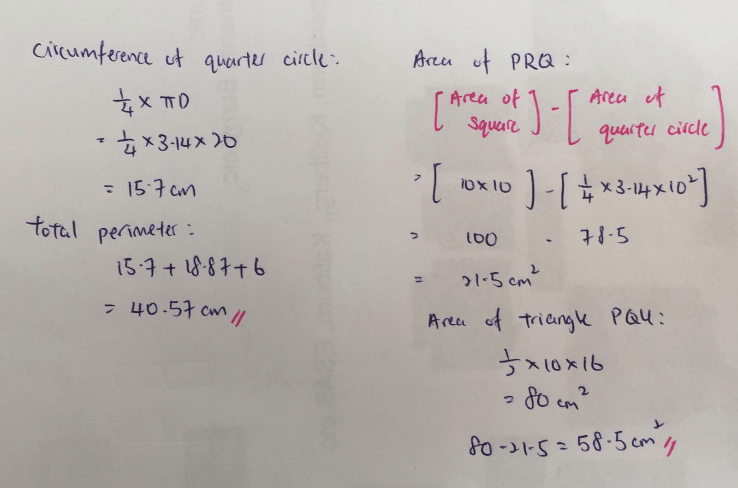# Question

perimeter of the shaded part = 18.87 + 6 + (1/4) x 3.14 x 10 x 2 = 40.57

area of the shaded part = (1/4) x 3.14 x 10 x 10 – (1/2) x 10 x 10 + (1/2) x 6 x 10 = 58.5

Ans : perimeter of the shaded part = 40.57 cm; area of the shaded part = 58.5 sq cm.

0 Replies 0 Likes0 Replies 0 Likes

This is a Geometry question.

 Quadrant Line Line Total Length 15.7 18.87 6 40.57

2 x 10 x 3.14 x 1/4 = 15.7

15.7 + 18.87 + 6 = 40.57 cm (Perimeter)

 Square Quadrant Boomerang Triangle Area 100 78.5 21.5 80

10 x 10 = 100

10 x 10 x 3.14 x 1/4 = 78.5

100 – 78.5 = 21.5

1/2 x 10 x 16 = 80

80 – 21.5 = 58.5 cm2

0 Replies 0 Likes